Bookmark

# Improving Math Skills

When you think of math, what do you think of? Adding numbers? Counting change? Measuring with a ruler? Dividing something into equal parts?

Math is this and much more. Learning math is almost like learning a new language. Math has its own special words and symbols. Math also has its own skills and strategies. This chapter will help you understand the language and strategies of mathematics. At the end of the chapter, you will find all kinds of helpful charts and tables.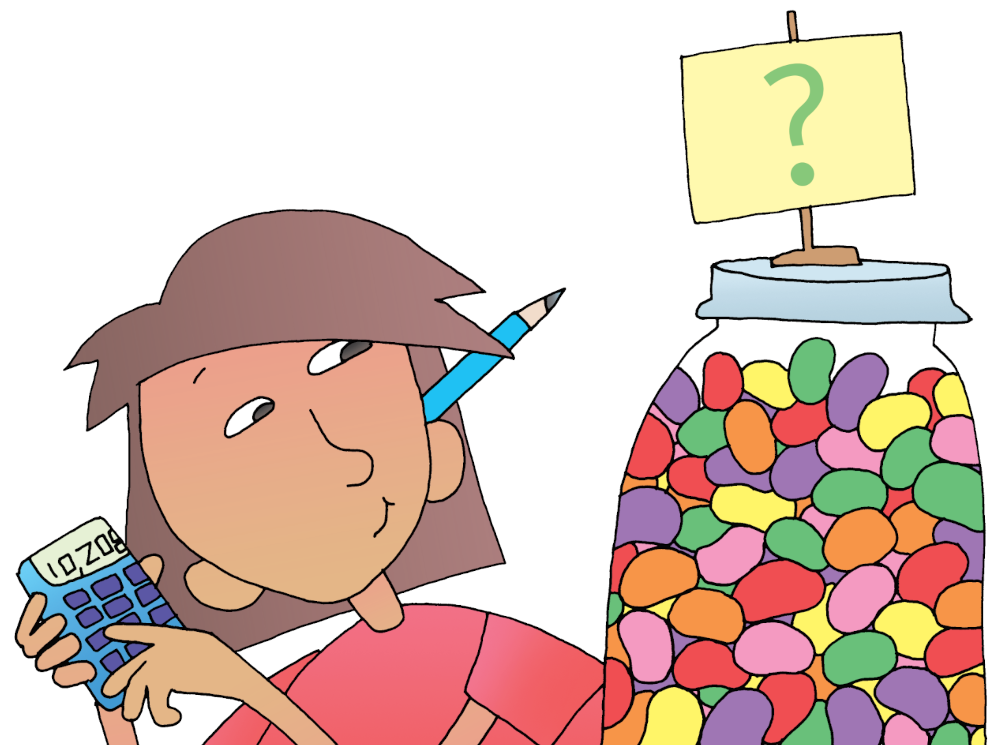# Solving Basic Word Problems

Most of your math assignments include at least two or three word problems. The best way to solve word problems is to follow a process. That way you won’t miss any important details. Here’s a process to try:

## Four-Step Process

Be sure you understand all the parts. Look for any key words like “how many” or “in all.”

### 2. Decide what you need to do.

Do you have to add a series of numbers? Do you have to multiply or subtract? Do you have to do more than one thing—maybe add, then subtract?

### 3. Solve the problem.

There may be more than one way to solve a problem. Page 393 shows you five strategies for figuring out the same problem. (Remember, show all of your work so you can check it later.)

Here are two ways to check: (1) You can do the problem again, only in a different way. (2) You can start with your answer and work backward. Let’s say that your answer to a problem is 42. You multiplied 6 times 7 to get that answer. If you divide 42 by 6, you should get 7. Or if you divide 42 by 7, you should get 6.

## Sample Word Problem

Susan and her best friend, Maria, are making chocolate cookies for their soccer picnic. There will be 13 people at the picnic, including four parents. Susan and Maria want to give each person three cookies. How many cookies do they need to make?

### 2. Decide what you need to do.

After reading the problem, you know that you are looking for a total number of cookies. You also know the basic numbers you have to work with: 13 people with 3 cookies each.

### 3. Solve the problem.

Let’s say you decide to skip-count by three, 13 times. (See the next page for other strategies.)

### 3, 6, 9, 12, 15, 18, 21, 24, 27, 30, 33, 36, 39

To check your work, you decide to solve the problem in a different way. You make tally marks in 13 groups of three (/// /// /// . . . ). You then add up all of your marks. (Your work checks out. The girls need to make 39 cookies.)

# Five Problem-Solving Strategies

There are many different ways to solve a word problem. Five different strategies are given below. (The numbers are from the sample word problem on page 392.)

### 13 people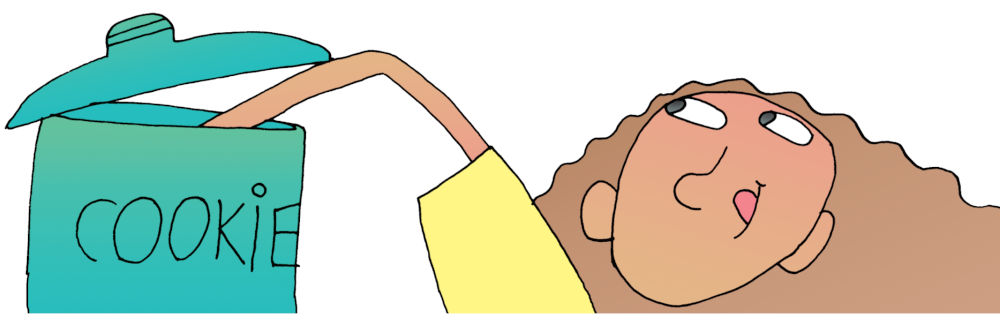# Solving Brain Stretchers

Sometimes you are asked to do problems that can’t be solved by simply multiplying or dividing the numbers. We call these types of problems brain stretchers. Your teacher may give you these problems to get you thinking. Here’s such a problem:

## Problem-Solving Strategy

Let’s see how one person works on this problem.

# Symbols, Numbers, and Tables

Use the following symbols, tables, and charts whenever you need help with your math.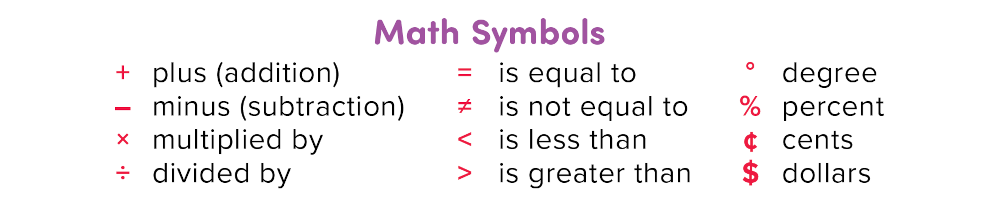To add two numbers (8 + 6), find one of the numbers at the beginning of a row (8). Find your second number at the top of a column (6). Find your answer where the row and column meet (8 + 6 = 14).

To subtract two numbers (14 – 6), locate the larger number in the table (14). Then subtract the number at the top of the column (6). Your answer will be in the row to the far left (8).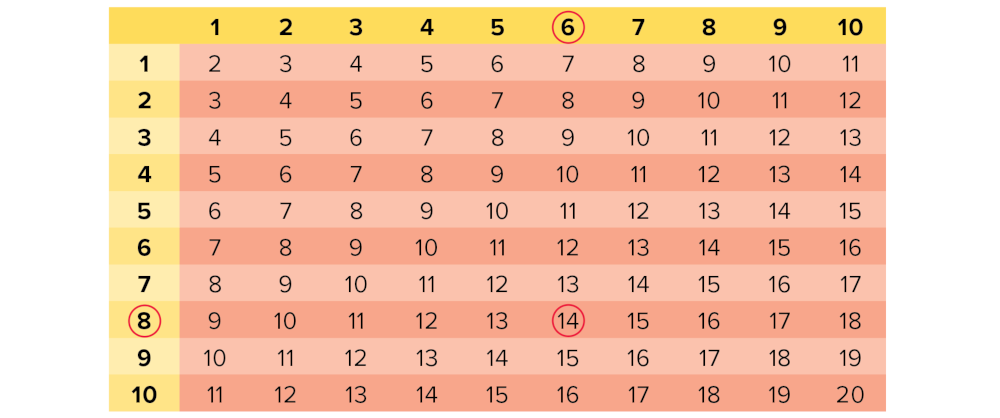## Multiplication and Division Table

To multiply two numbers (4 × 6), find one of the numbers at the top of a column (4). Find the second number at the beginning of a row (6). Find your answer where the row and column meet (6 × 4 = 24). To divide (24 ÷ 6), find the larger number in the table (24). Then divide by the number at the far left (6). Your answer is at the top of the column (4).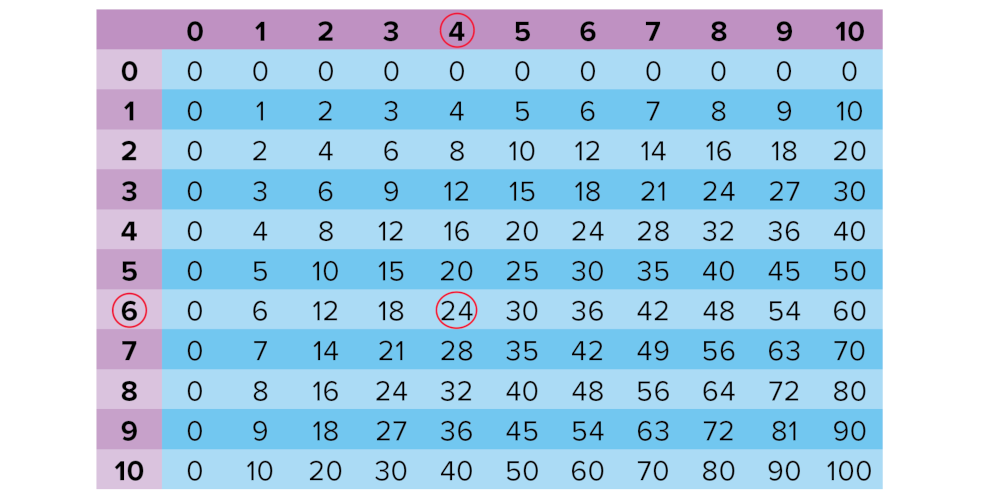## Roman Numerals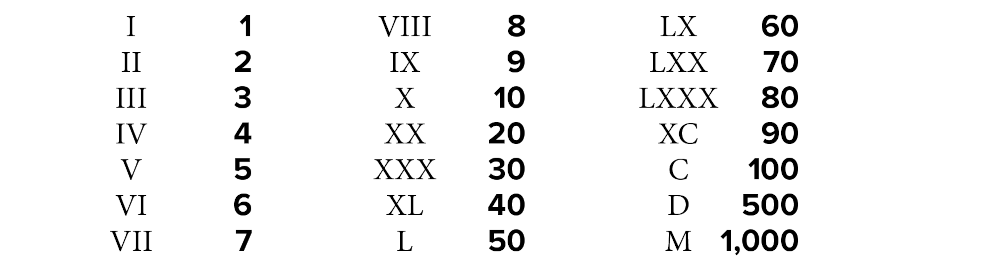## Rounding Numbers

You can round a number to the nearest ten, hundred, thousand, or even million.

### Rounding to the Nearest Ten

If your number is 32, 32 is closer to 30 than to 40. So, 32 rounded to the nearest ten is 30.The number 36 is closer to 40 than to 30. So, 36 rounded to the nearest ten is 40.Numbers ending in 1, 2, 3, or 4 are rounded down. Those ending in 6, 7, 8, or 9 are rounded up. And even though 5 is right in the middle, it is rounded up. So, 35 rounded to the nearest ten is 40.## Skip-Counting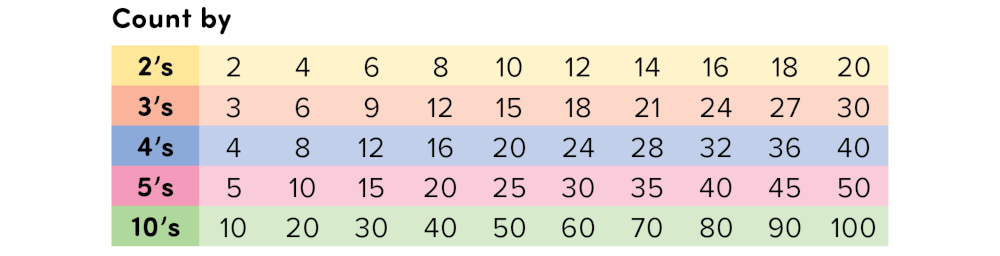# Place-Value Chart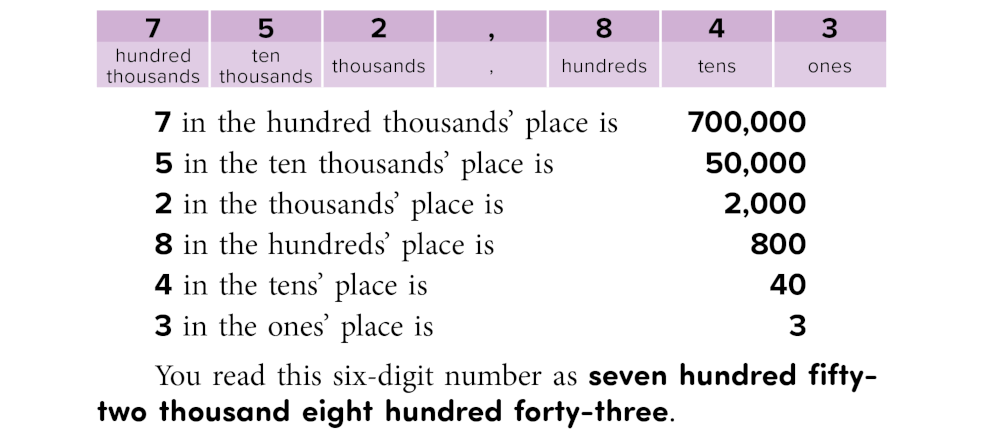# Fractions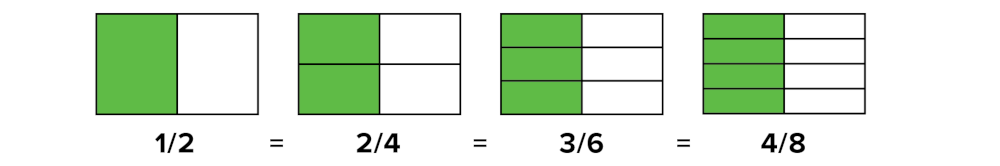In 1/2, 1 is the numerator and 2 is the denominator. All the fractions above are called equivalent fractions. They all name the same part, or fraction, of the square . . . even though they have different numerators and denominators. Fractions with the same denominators are easy to compare.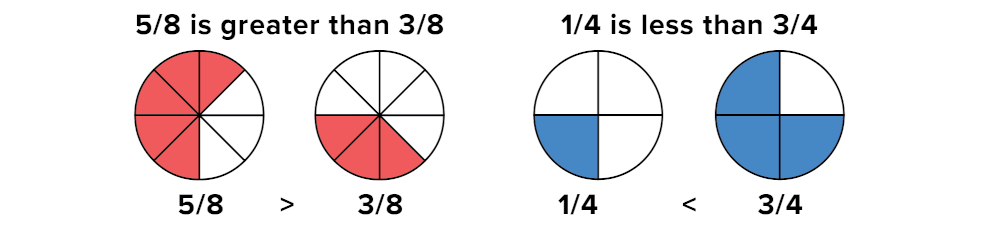## WT 399

### Page 399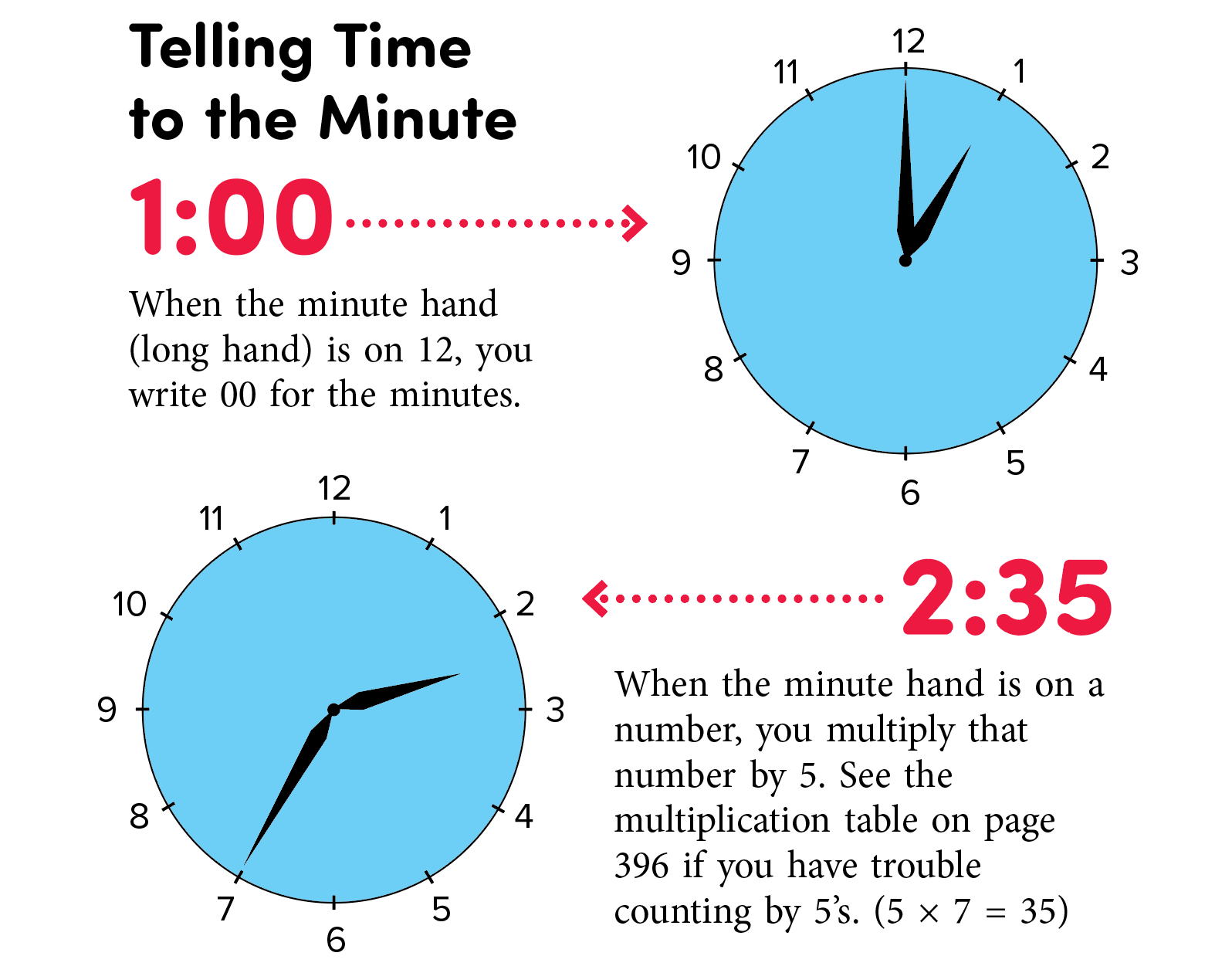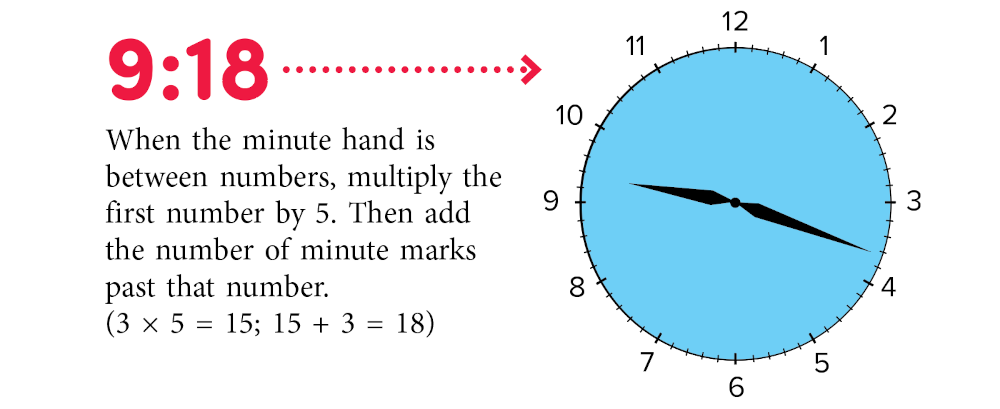Current Page

Level:

## Standards Correlations:

The Common Core State Standards provide a way to evaluate your students' performance.

## Lesson Plan Resources:

Here you'll find a full list of resources found in this lesson plan.

Vocabulary List:
• math: system for using numbers

Vocabulary List:
• word problem: story that provides mathematical questions that require answers

Vocabulary List:
• skip-counting: counting by twos, threes, fours, or other numbers

• tally marks: small slashes that you can count

Vocabulary List:
• brain stretcher: problem that requires critical thinking

© 2023 Thoughtful Learning. Copying is permitted.

k12.thoughtfullearning.com